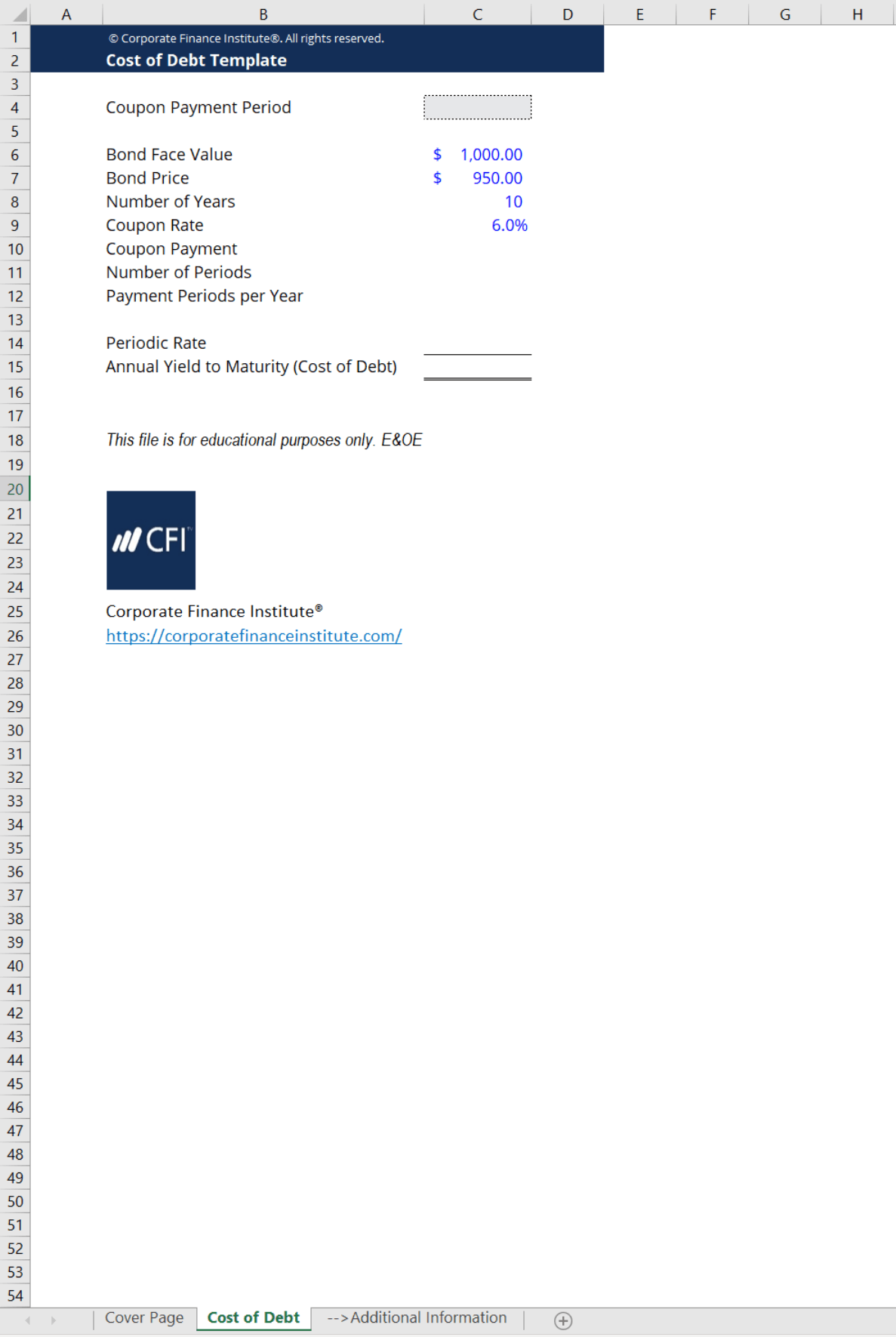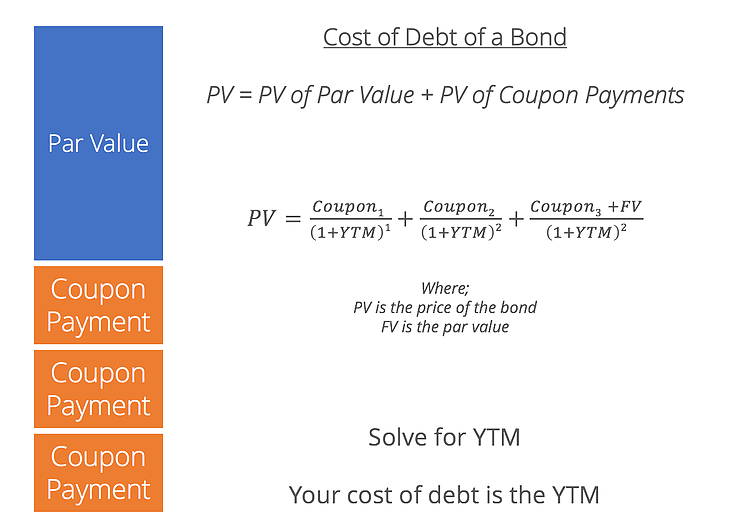# Cost of Debt Calculator

## Cost of Debt Calculator

This cost of debt calculator is used to calculate the annual yield to maturity of a company’s debt, otherwise known as its cost of debt or the interest rate. This calculator takes the following values for its inputs:

• bond face value
• bond price
• number of years to maturity
• coupon payment period (e.g. monthly, quarterly, etc.)
• coupon rate

Here is a preview of the cost of debt calculator:### Estimating Cost of Debt

The cost of debt is the return provided to the debtholders and creditors of a company. These investors are given a return to compensate for the risk that comes with lending money.

Observable interest rates often contribute to quantifying cost of debt. Therefore, the cost of debt of a company reflects both its risk of default as well as the interest rates in the market. Additionally, it is also a component in calculating Weighted Average Cost of Capital (WACC).

There are two methods to calculating cost of debt:

1. Calculating the yield to maturity (YTM) of a company’s debt
2. Determining the cost of debt by referencing the credit rating of the firm

This cost of debt calculator uses the first calculation method. If a company is public, it can have observable debt in the market. We can look at the company’s bonds and use the values mentioned above to solve for the YTM of the bond. It is best to use this method when the company you are looking at has a simple capital structure. If it has a more complicated capital structure with multiple tranches of debt, there will be multiple differing interest rates.### More Free Templates

For more resources, check out our business templates library to download numerous free Excel modeling, PowerPoint presentation and Word document templates.

• Excel Modeling Templates
• PowerPoint Presentation Templates
• Transaction Document Templates
• CFI Template Marketplace

### Financial Analyst Certification

Become a certified Financial Modeling and Valuation Analyst (FMVA)® by completing CFI’s online financial modeling classes and training program!